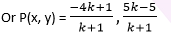Newbie

# Find the ratio in which the line segment joining A (1, – 5) and B (- 4, 5) is divided by the x-axis. Also find the coordinates of the point of division. Q.5

• 0

What is the answer of coordinate geometry of class 10th  , how i solve this question fastly and what is the best solution of this question . Find the ratio in which the line segment joining A (1, – 5) and B (- 4, 5) is divided by the x-axis. Also find the coordinates of the point of division.

Share

1. Let the ratio in which the line segment joining A (1, – 5) and B ( – 4, 5) is divided by x-axis be k : 1. Therefore, the coordinates of the point of division, say P(x, y) is ((-4k+1)/(k+1), (5k-5)/(k+1)).We know that y-coordinate of any point on x-axis is 0.

Therefore, ( 5k – 5)/(k + 1) = 0

5k = 5

or k = 1

So, x-axis divides the line segment in the ratio 1:1.

Now, find the coordinates of the point of division:

P (x, y) = ((-4(1)+1)/(1+1) , (5(1)-5)/(1+1)) = (-3/2 , 0)

• 0